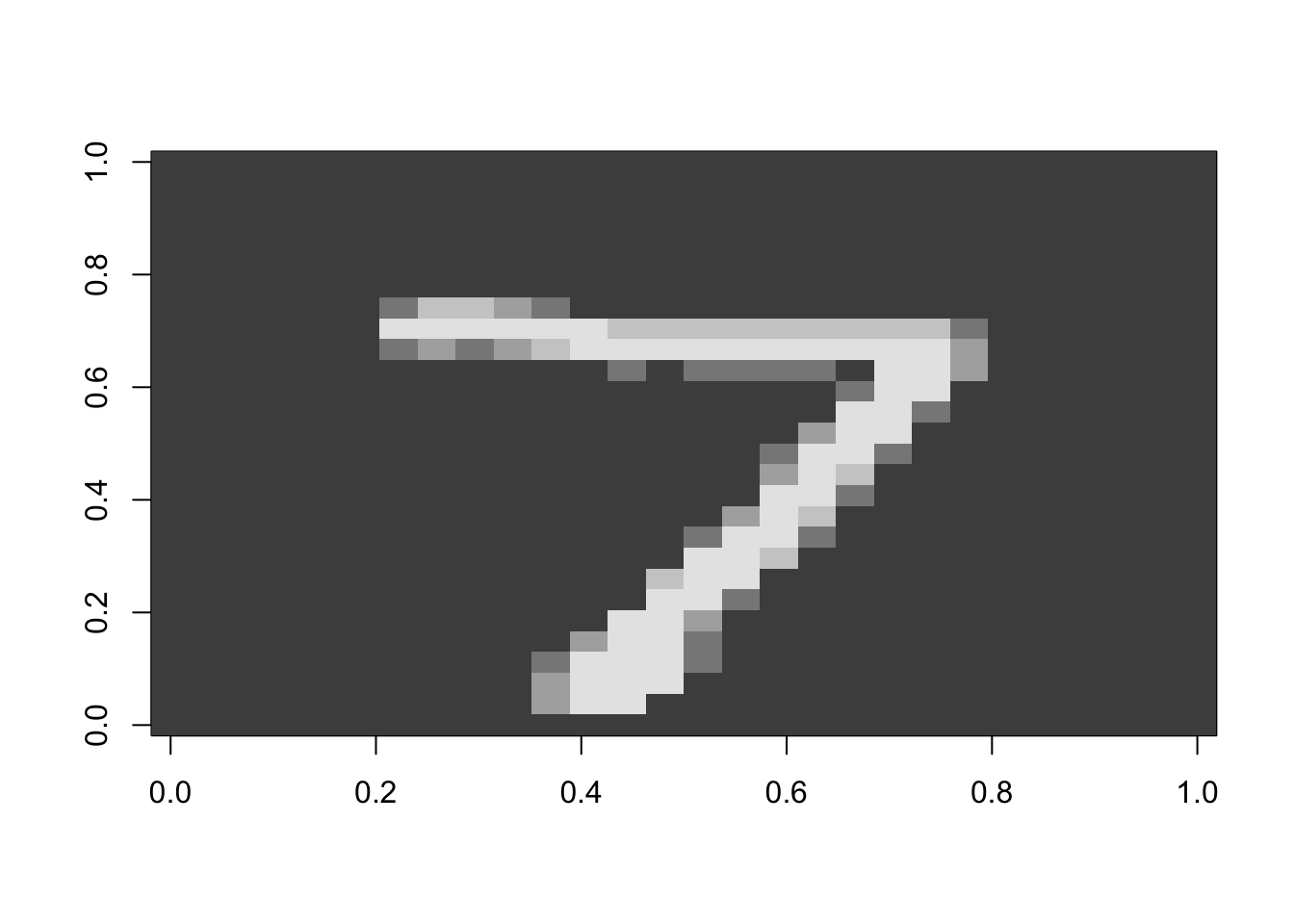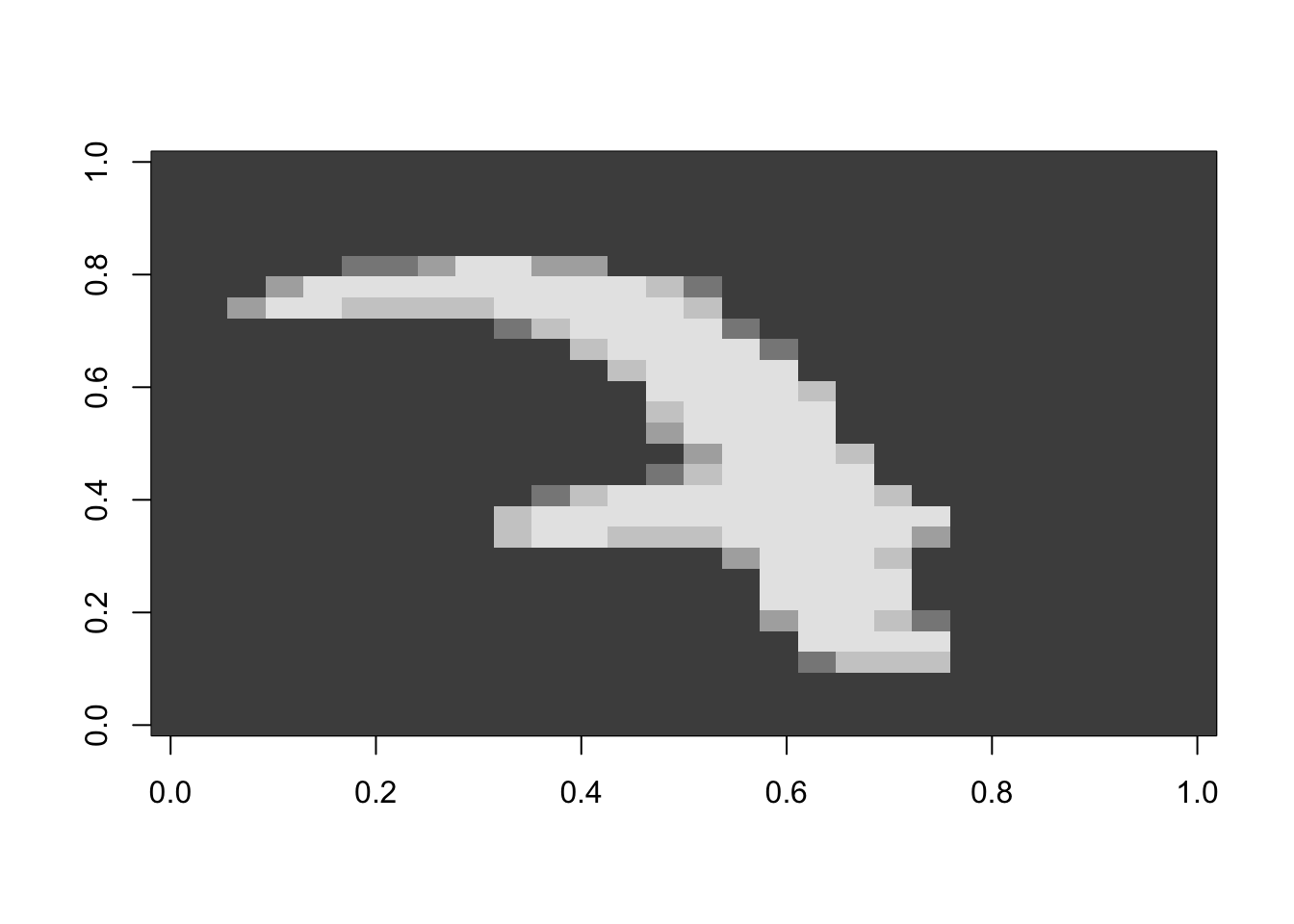### What do we need

  library(magrittr)
library(keras)

Installing keras requires

• Python (2 for CPU, 3 for GPU).

• A virtual environment (virtualenv, anaconda)

• Tensorflow is the default option

install_keras(tensorflow = "default")
• Recommended R package: magrittr

### A MNIST example

• The MNIST database is a large database of handwritten digits that is commonly used for training various image processing systems.

• Handwritten digits are stored as squared matrices of size 28 by 28 (784) pixels. Each pixel has 256 values.

  mnist <- dataset_mnist()
x_train <- mnist$train$x
y_train <- mnist$train$y
x_test <- mnist$test$x
y_test <- mnist$test$y
• Question: Discriminate digit 7 ($$y = 1$$) from digit 2 ($$y = 0$$)
# extract digits 2 and 7 from MNIST and build some training data
boo_train <- y_train == 2 | y_train == 7
x_train <- mnist$train$x[boo_train,,]
y_train <- mnist$train$y[boo_train]

# same for test data
boo_test <- y_test == 2 | y_test == 7
x_test <- mnist$test$x[boo_test,,]
y_test <- mnist$test$y[boo_test]
• Most “7” images look like this one (Note that french people write them differently).
  image(t(x_test[1, 28:1,]), col = grey.colors(5))• We need to flatten the images (= convert them as vectors) and to rescale their values
# Flattening
x_train <- array_reshape(x_train,
c(nrow(x_train),
784))
x_test <- array_reshape(x_test,
c(nrow(x_test),
784))

# Rescaling
x_train <- x_train/255
x_test <- x_test/255
• Convert the labels {2,7} into binary values
  y_train <- as.numeric(y_train == 7)
y_test <-  as.numeric(y_test == 7)

### Building models with keras

• It is based on functional programming. Consider a single hidden layer with 128 neurons. Half of the units are dropped out during an epoch.
model <- keras_model_sequential()
model %>%
layer_dense(units = 128,
activation = 'relu',
input_shape = 784) %>%
layer_dropout(rate = 0.5) %>%
layer_dense(units = 1,
activation = 'sigmoid')
• Compiling models with keras. We need to specify a loss function and a minimization method
model %>% compile(
loss = 'binary_crossentropy',
optimizer = optimizer_sgd(lr = 0.01,
decay = 0.001),
metrics = c('accuracy')
)
• Fitting our neural network model
set.seed(110101011)
history <- model %>%
fit(x_train,
y_train,
epochs = 20,
batch_size = 100,
validation_data = list(x_test, y_test),
verbose = 0
)

### Evaluating the results

• Log-loss and accuracy
model %>% evaluate(x_test, y_test)
## $loss ##  0.08389094 ## ##$acc
##  0.9742718
• Confusion matrix
pred_class <- model %>%
predict_classes(x_test)

table(predicted = pred_class,
observed = mnist$test$y[boo_test])
##          observed
## predicted    2    7
##         0 1015   36
##         1   17  992
• Let see an example of a true “two” misclassified as a “seven”.
false_positive <- which(mnist$test$y[boo_test] == 7 & pred_class[,1] == 0)
x_test[false_positive,] %>% matrix(nrow = 28) %>% .[,28:1] %>% image(col = grey.colors(5))### For fun: using pretrained models

• Using pretrained model is one of the most common applications of DL.
pretrained_model = application_resnet50(weights = 'imagenet')

Let’s consider our misclassified example.

img <- mnist$test$x[false_positive,,]

x <- array(NA, c(1,28,28,3))
x[1, , ,1:3] <- img
x <- image_array_resize(x, 224, 224)
x <- imagenet_preprocess_input(x)

And see it’s prediction in Imagenet (yes, that’s n’imp)

pred <- pretrained_model %>% predict(x)
imagenet_decode_predictions(pred, top = 4)[]